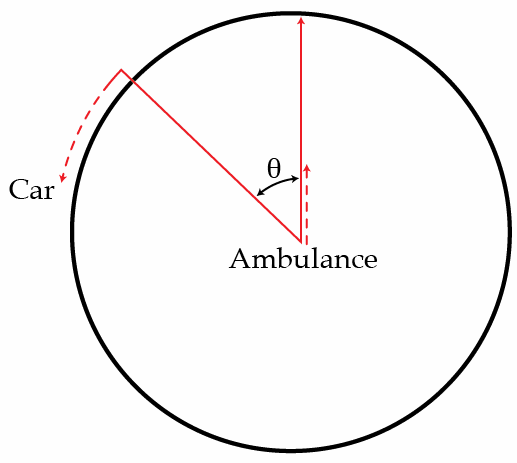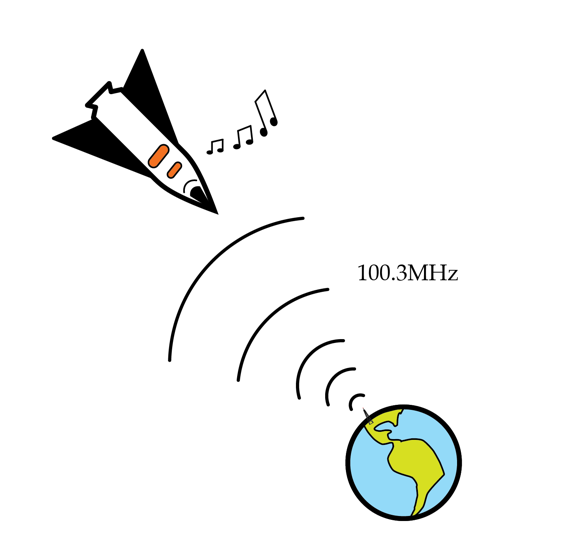Classical Mechanics

# Waves: Level 3-5 Challenges

A boy is standing in front of a stationary train. The train blows a horn of frequency 400 Hz. If the wind is blowing from the train to the boy at a speed of 30 m/s, what will be the frequency of the sound the boy hears?Recordings that get played speakers can be very detailed, consisting of tens or hundred of distinct sound sources, each of which contribute a continuum of frequency components. Yet there is only one speaker. How is it possible for a single speaker to faithfully reproduce complex sounds?

An ambulance car is going with a speed of $60~\mbox{km/h}$, while a car is trying to go around it in a circle with a speed $50~\mbox{km/h}$. If the sound that ambulance car emits has a frequency of $1~\mbox{kHz}$, which frequency does the driver of the car hear in Hz when the ambulance car is in the center of the circle it makes, and the car makes an angle $\theta = 30^\circ$ with the direction of the car?Details and assumptions

• Speed of sound in the air is $c = 1235~\mbox{km/h}$.

I'm in a spaceship very far away from Earth but traveling straight towards earth with a speed v. It's boring out here, so I decide to try and tune in to some of my favorite earthly radio stations. I remember that my favorite station has a frequency of 100.3 MHz and so tune my radio to exactly this frequency. Amazingly, I hear the radio station just like I do on Earth! How fast is my spaceship going in m/s? (Hint: it's not that fast... I think I should check whether my engines are on).Details and assumptions

• Photons of electromagnetic radiation have an intrinsic kinetic energy related to their frequency by $E=hf$ where $h$ is Planck's constant.
• The gravitational interaction between photons and Earth can be treated via usual Newtonian gravity and $E=mc^2$ to convert between energy and mass.
• The total energy of the photons is conserved.
• The Earth can be treated as a sphere of radius 6370 km and mass $6 \times 10^{24}~kg$. You can ignore rotation of the earth.
• The speed of light is $3 \times 10^8~m/s$.
• Assume the earth is at rest.

Airplanes are noisy things. For example, if you stand really close to a runway, a military jet can produce a sound intensity of about $100\text{ W/m}^2$ at a distance of 30 m from the plane (this is beyond painful noise). You live near a military airport and want to work on physics with your friend. Since you don't want to shout, you decide to find a spot far enough away from the airport that the airplane noise is below the threshold of conversation $(10^{-6}\text{ W/m}^2)$. How far do you need to go from the airport in kilometers to accomplish this?

×